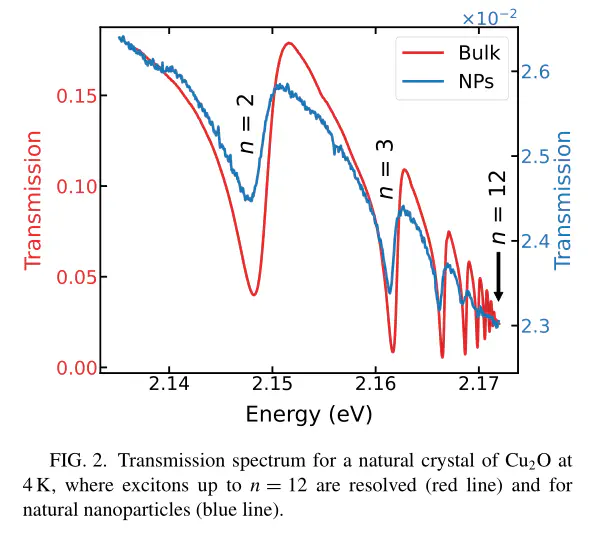# Quantum confined Rydberg excitons in Cu2O nanoparticles### Abstract

The quantum confinement of Rydberg excitons is an important step towards exploiting their large nonlinearities for quantum applications. We observe Rydberg excitons in natural nanoparticles of Cu$_2$O. We resolve up to the principal quantum number $n=12$ in a bulk Cu$_2$O crystal and up to $n=6$ in nanoparticles extracted from the same crystal. The exciton transitions in nanoparticles are broadened and their oscillator strengths decrease as $\propto n^{-4}$ compared to those in the bulk (decreasing as $\propto n^{-3}$). We explain our results by including the effect of quantum confinement of exciton states in the nanoparticles. Our results provide an understanding of the physics of Cu$_2$O Rydberg excitons in confined dimensions.

Publication
Physical Review B